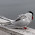## Monday, June 16, 2014

### Converting Coordinates between map projections using Python

Python can be used very nicely to quickly convert coordinates from one projection into another using the pyproj package, as usual available here. This example does it from command line, but you can built it into your script, of course, as well. The easiest way is to find the EPSG code for your projections, for example on this page. You then initialize the projections you want, in this example unprojected lat/long into EPSG:3575:

```>>> EPSG4326 = pyproj.Proj("+init=EPSG:4326")
>>> EPSG3575 = pyproj.Proj("+init=EPSG:3575")
```

Alternatively you can use the Proj.4 definition, so the following two lines are doing the same, you can use either of them
```EPSG3995 = pyproj.Proj("+proj=stere +lat_0=90 +lat_ts=71 +lon_0=0 +k=1 +x_0=0 +y_0=0 +datum=WGS84 +units=m +no_defs ")
EPSG3995 = pyproj.Proj("+init=EPSG:3995")
```

Now you can define the input coordinates and transform them, in this example you transform values from EPSG:4326 to EPSG:3575:

```>>> lat = 79
>>> long = -5
>>> x, y = pyproj.transform(EPSG4326, EPSG3575, long, lat)
>>> x,y
(-317466.6103301885, -1184801.519458934)
```

Official documentation can be found here

1.2.